## How to Calculate and Solve for Reaction: Lift Moves Down | Motion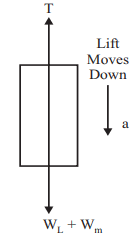The image above represents reaction: lift moves down.

To compute for reaction: lift moves down, three essential parameters are needed and these parameters are mass (m), acceleration (a) and acceleration due to gravity (g).

The formula for calculating reaction: lift moves down:

R = m(g – a)

Where;

R = Reaction
m = Mass
g = acceleration due to gravity
a = Acceleration

Let’s solve an example;
Find the reaction when the mass is 28, acceleration is 9 and acceleration due to gravity is 9.8.

This implies that;

m = Mass = 28
g = acceleration due to gravity = 9.8
a = Acceleration = 9

R = m(g – a)
R = 28(9.8 – 9)
R = 28(0.80)
R = 22.4

Therefore, the reaction is 22.4 N.

Calculating the Mass when the Reaction, the Acceleration and the Acceleration due to Gravity is Given.

m = R / g – a

Where;

m = Mass
R = Reaction
g = acceleration due to gravity
a = Acceleration

Let’s solve an example;
Find the mass when the reaction is 42, the acceleration is 8 and the acceleration due to gravity is 9.8

This implies that;

R = Reaction = 42
g = acceleration due to gravity = 9.8
a = Acceleration = 8

m = R / g – a
m = 42 / 9.8 + 8
m = 42 / 17.8
m = 2.359

Therefore, the mass is 2.359.

## How to Calculate and Solve for Reaction: Lift Moves Up | Motion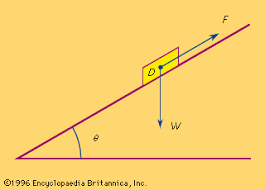The image above represents reaction: lift moves up.

To compute for reaction: lift moves up, three essential parameters are needed and these parameters are mass (m), acceleration (a) and acceleration due to gravity (g).

The formula for calculating reaction: lift moves up:

R = m(a + g)

Where;

R = Reaction
m = Mass
g = acceleration due to gravity
a = Acceleration

Let’s solve an example;
Find the reaction when the mass is 18, acceleration is 21 and acceleration due to gravity is 9.8.

This implies that;

m = Mass = 18
g = acceleration due to gravity = 21
a = Acceleration = 9.8

R = m(a + g)
R = 18(21 + 9.8)
R = 18(30.8)
R = 554.4

Therefore, the reaction is 554.4 N.

Calculating the Mass when the Reaction, the Acceleration and the Acceleration due to Gravity is Given.

m = R / a + g

Where;

m = Mass
R = Reaction
g = acceleration due to gravity
a = Acceleration

Let’s solve an example;
Find the mass when the reaction is 42, the acceleration is 21 and the acceleration due to gravity is 9.8

This implies that;

R = Reaction = 42
g = acceleration due to gravity = 9.8
a = Acceleration = 21

m = R / a + g
m = 42 / 21 + 9.8
m = 42 / 30.8
m = 1.36

Therefore, the mass is 1.36.

## How to Calculate and Solve for Force | Motion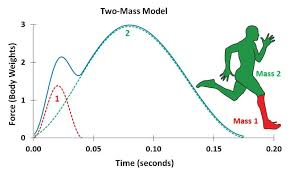The image above represents force.

To compute for force, two essential parameters are needed and these parameters are mass (m) and acceleration (a).

The formula for calculating force:

F = ma

Where;

F = Force
m = Mass
a = Acceleration

Let’s solve an example;
Find the force when the mass is 12 and the acceleration is 26?

This implies that;

m = Mass = 12
a = Acceleration = 26

F = ma
F = 12 x 26
F = 312

Therefore, the force is 312 N.

Calculating for Mass when the Force and the Acceleration is Given.

m = F / a

Where;

m = Mass
F = Force
a = Acceleration

Let’s solve an example;
Find the mass when the force is 100 and the acceleration is 20.

This implies that;

F = Force = 100
a = Acceleration = 20

m = F / a
m = 100 / 20
m = 5

Therefore, the mass is 5.

## How to Calculate and Solve for Final Velocity | Motion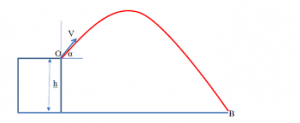The image above represents final velocity.

To compute for final velocity, three essential parameters are needed and these parameters are initial velocity (u), acceleration (a) and time (t).

The formula for calculating final velocity:

v = u + at

Where;

v = Final Velocity
u = Initial Velocity
a = Acceleration
t = Time

Let’s solve an example;
Find the Final velocity when the initial velocity is 12, acceleration is 9 and the time is 24.

This implies that;

u = Initial Velocity = 12
a = Acceleration = 9
t = Time = 24

v = u + at
v = 12 + (9 x 24)
v = 12 + 216
v = 228

Therefore, the final velocity is 228 m/s.

Calculating the Initial Velocity when the Final Velocity, the Acceleration and the Time is Given.

u = v – at

Where;

u = Initial Velocity
v = Final Velocity
a = Acceleration
t = Time

Let’s solve an example;
Find the Initial Velocity when the Final Velocity is 32, the acceleration is 12 and the time is 2.

This implies that;

v = Final Velocity = 32
a = Acceleration = 12
t = Time = 2

u = v – at
u = 32 – (12 x 2)
u = 32 – 24
u = 8

Therefore, the initial velocity is 8 m/s.

## How to Calculate and Solve for Acceleration | Motion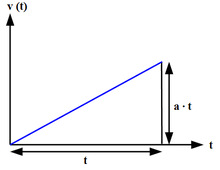The image above represents acceleration.

To compute for acceleration, three essential parameters are needed and these parameters are initial velocity (u), final velocity (v) and time (t).

The formula for calculating acceleration:

a = (v – u) / t

Where;

a = Acceleration
v = Final Velocity
u = Initial Velocity
t = Time

Let’s solve an example;
Find the acceleration when the final velocity is 21, the initial velocity is 10 and time is 8.

This implies that;

v = Final Velocity =21
u = Initial Velocity = 10
t = Time = 8

a = (v – u) / t
a = (21 – 10) / 8
a = 11 / 8
a = 1.375

Therefore, the acceleration is 1.375 m/s².

Calculating the Initial Velocity when the Acceleration, the Final Velocity and the Time is Given.

u = v – at

Where;

u = Initial Velocity
a = Acceleration
v = Final Velocity
t = Time

Let’s solve an example;
Find the initial velocity when the acceleration is 14, the final velocity is 34 and the time is 2.

This implies that;

a = Acceleration = 14
v = Final Velocity = 34
t = Time = 2

u = v – at
u = 34 – (14 x 2)
u = 34 – 28
u = 6

Therefore, the initial velocity is 6 m/s.

## How to Calculate and Solve for Distance Covered | Motion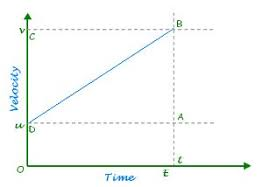The image above represents distance covered.

To compute for distance covered, three essential parameters are needed and these parameters are initial velocity (u), acceleration (a) and time (t).

The formula for calculating distance covered:

S = ut + 0.5at²

Where;

S = Distance Covered
u = Initial Velocity
t = Time
a = Acceleration

Let’s solve an example;
Find the distance covered when the initial velocity is 21, acceleration is 10 and the time is 15.

This implies that;

u = Initial Velocity = 21
t = Time = 15
a = Acceleration = 10

S = ut + 0.5at²
S = (21 x 15) + (0.5 x 10 x 15²)
S = 315 + (0.5 x 10 x 225)
S = 315 + (1125)
S = 1440

Therefore, the distance covered is 1440 m .

Calculating the Initial Velocity when the Distance Covered, the Acceleration and the Time is Given.

u = S – 0.5at2 / t

Where;

u = Initial Velocity
S = Distance Covered
t = Time
a = Acceleration

Let’s solve an example;
Find the initial velocity when the distance covered is 48 with a time of 8 and an acceleration of 9.

This implies that;

S = Distance Covered = 48
t = Time = 8
a = Acceleration = 9

u = S – 0.5at2 / t
u = 48 – 0.5(9)(82) / 8
u = 48 – 0.5(9)(64) / 8
u = 48 – 288 / 8
u = – 240 / 8
u = – 30

Therefore, the initial velocity is – 30 m/s.

## How to Calculate and Solve for Final Velocity | Motion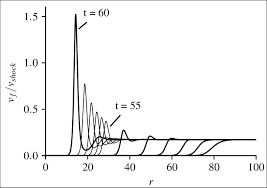The image above represents final velocity.

To compute for final velocity, three essential parameters are needed and these parameters are initial velocity (u), acceleration (a) and distance covered (S).

The formula for calculating final velocity:

v = √(u² + 2as)

Where;

v = Final Velocity
u = Initial Velocity
a = Acceleration
s = Distance Covered

Let’s solve an example;
Find the final velocity when the initial velocity is 13, acceleration is 9.8 and the distance covered is 32.

This implies that;

u = Initial Velocity = 13
a = Acceleration = 9.8
s = Distance Covered = 32

v = √(u² + 2as)
v = √(13² + (2 x 9.8 x 32))
v = √(169 + (627.2))
v = √(796.2)
v = 28.21

Therefore, the final velocity is 28.21 m/s.

Calculating the Initial Velocity when the Final Velocity, the Acceleration and the Distance Covered is Given.

u = √v² – 2as

Where;

u = Initial Velocity
v = Final Velocity
a = Acceleration
s = Distance Covered

Let’s solve an example;
Find the initial velocity when the final velocity is 30, the acceleration is 8 and the distance covered is 11.

This implies that;

v = Final Velocity = 30
a = Acceleration = 8
s = Distance Covered = 11

u = √v² – 2as
u = √30² – 2(8 x 11)
u = √900 – 2(88)
u = √900 – 176
u = √724
u = 26.90

Therefore, the initial velocity is 26.90 m/s.